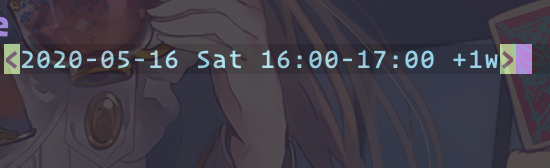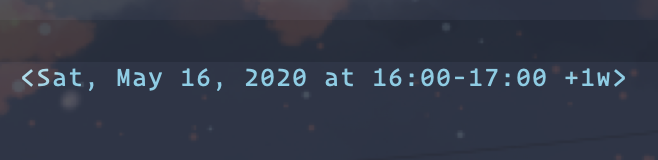# org mode 输入时间时，如何同时输入重复间隔 （比如 +1w）repeater interval

C-c . RET C-b SPC +1w------- Edit -----

https://orgmode.org/manual/The-date_002ftime-prompt.html

Emacs 内置的《Intro to Emacs Lisp》里有答案

`'("<%a, %b %d, %Y>" . "<%a, %b %d, %Y at %H:%M>")```````  (when repeater
(backward-char)
(insert " " repeater)
``````

``````  (let* ((ts (cond
((org-at-date-range-p t)
(match-string (if (< (point) (- (match-beginning 2) 2)) 1 2)))
((org-at-timestamp-p 'lax) (match-string 0))))
.....
(repeater (and ts
(string-match "\\([.+-]+[0-9]+[hdwmy] ?\\)+" ts)
(match-string 0 ts)))
``````

``````(define-advice org-time-stamp (:around (fn &rest args) insert-escaped-repeater)
;;---------------------------
;; C-c . \+1w RET ;; => <2020-05-23 Sat +1w>
;; C-c . \-1w RET ;; => <2020-05-23 Sat -1w>
;;---------------------------
(apply fn args)
(save-excursion
(backward-char)
(insert " "
(string-trim-right
``````

6赞

``````(defun hello ()
(interactive)
(let((org-time-stamp-formats
'("<%Y-%m-%d %a +1w>" . "<%Y-%m-%d %a %H:%M +1w>"))
)
(call-interactively 'org-time-stamp)
))
``````

repeater依赖于ts 也就是说你得先输入 1w 才能得到

``````(repeater
(or
(and
(org-string-nw-p time)
(string-match org-repeat-re time)
(match-string 1 time))
(and
(org-string-nw-p old-date)
(string-match "\\([.+-]+[0-9]+[hdwmy]\\(?:[/ ][-+]?[0-9]+[hdwmy]\\)?\\)" old-date)
(match-string 1 old-date))))
``````

`(xr org-repeat-re)` 得到

``````(seq "<" (= 4 (any "0-9")) "-" (any "0-9") (any "0-9") "-" (any "0-9") (any "0-9") " " (*\? (not (any "
>"))) (group (opt (any "+.")) "+" (one-or-more (any "0-9")) (any "dhmwy") (opt (group "/" (one-or-more (any "0-9")) (any "dhmwy")))))
``````